# Radioactivity | Form Four Physics

### Guest Account

Attempt Form Four Physics Questions
Guest Account

- Radioactivity was discovered by Henri Becquerel in 1869. In 1898, Marie and Pierre Curie succeeded in chemically isolating two radioactive elements, Polonium (z=84) and Radium (z=88).
Radioactivity or radioactive decay is the spontaneous disintegration of unstable nuclides to form stable ones with the emission of radiation.
- Unstable nuclides continue to disintegrate until a stable atom is formed.
Alpha (α) and beta (β) particles are emitted and the gamma rays (γ) accompany the ejection of both alpha and beta particles.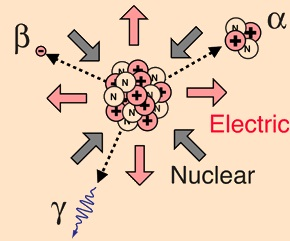## The nucleus

- The nucleus is made up of protons and neutrons.
- They are surrounded by negatively charged ions known as electrons.
- The number of protons is equal to the number of electrons.
- Both protons and neutrons have the same mass. The weight of an electron is relatively small compared to neutrons and protons. The number of protons in an atom is referred to as the proton number (atomic number) and denoted by the symbol Z.
- The number of neutrons is denoted by the symbol N.
- Protons and neutrons are called nucleons since they form the nucleus of an atom.
- The sum of both the protons and neutrons is called the mass number (A) or nucleon number
. Therefore:
A = Z + N and N = A - Z.

- The masses of atoms are conveniently given in terms of atomic mass units (u) where (u) is 1/12th the mass of one atom of carbon-12 and has a value of 1.660 × 10-27 kg.
- Hence the mass of one proton is equal to 1.67 × 10-27 and is equal to 1u.

Isotopes are elements with different mass numbers but with equal atomic numbers i.e. uranium with mass numbers 235 and 238.

#### a) Alpha (α) Particles

They are represented as 42He, hence with a nucleus number 4 and a charge of +2.
Properties
1. Their speeds are 1.67 × 107 m/s, which is 10% the speed of light.
2. They are positively charged with a magnitude of a charge double that of an electron.
3. They cause intense ionization hence loosing energy rapidly hence they have a very short range of about 8 cm in air.
4. They can be stopped by a thin sheet of paper, when stopped they capture two electrons and become helium gas atoms
5. They can be affected by photographic plates and produce flashes when incident on a fluorescent screen and produce heating effect in matter.
6. They are slightly deflected by a magnetic field indicating that they have comparatively large masses.

#### b) Beta (β) Particles

They are represented by ############################# meaning that they have no mass but a charge of -1.
Properties
1. Their speeds are as high as 99.9% or more the speed of light
2. They are deflected by electric and magnetic fields but in a direction opposite to that of alpha particles.
3. Due to their high speed they have a higher penetrative rate than alpha particles (about 100 times more)
4. They can be stopped by a thin sheet of aluminium
5. Their ionization power is much less intense about 1/100th that of alpha particles.

#### c) Gamma (γ) Particles

They have very short wavelengths in the order of 10-10 m and below.
Properties
1. They travel at the speed of light.
2. They have less ionization power than that of both alpha and beta particles
3. They accompany the emission of alpha and beta particles
4. They carry no electric charge hence they are not deflected by both electric and magnetic fields.
5. They have more penetrating power than X-rays.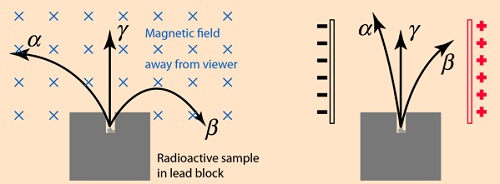#### 1. Gold Leaf Electroscope

- The rate of collapse of the leaf depends on the nature and intensity of radiation.
- The radioactive source ionizes the air around the electroscope.
- Beta particles discharges a positively charged electroscope with the negative charge neutralizing the charge of the electroscope. Alpha particles would similarly discharge a negatively charged electroscope.
- To detect both alpha and beta particles a charged electroscope may not be suitable because their ionization in air may not be sufficiently intense making the leaf not to fall noticeably.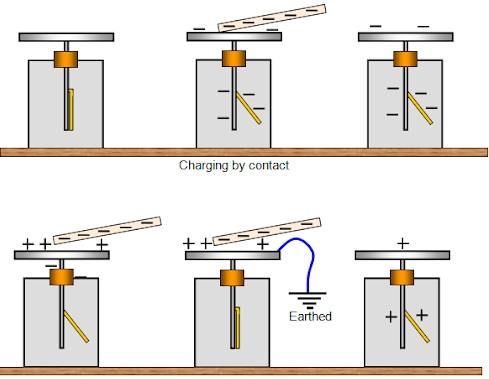#### 2. The Spark Counter

- The detector is shown belowThis detector is suitable for alpha sources due to the inadequacy of the ionization by both beta and gamma radiations. By putting the source away from the gauze or placing a sheet of paper between the two one can determine the range and penetration of the alpha particles.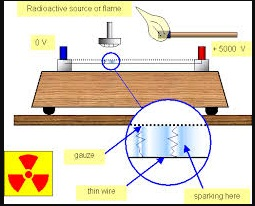#### 3. Geiger Muller (GM) Tube

- It is illustrated as below #################
- The mica window allows passage of alpha, beta and gamma radiations.
- The radiations ionize the gas inside the tube. The electrons move to the anode while the positive ions move to the cathode.
- As the ions are produced there are collisions which produce small currents which are in turn amplified and passed to the scale. The scale counts the pulses and shows the total on a display screen.
- After each pulse the gas returns to normal ready for the next particle to enter.
- A small presence of halogen gas in the tube helps in absorbing the positive ions to reduce further ionization and hence a quick return to normal. This is called quenching the tube.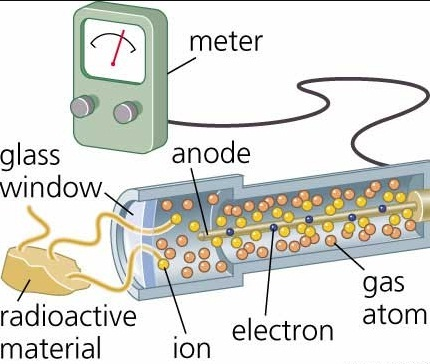#### 4. The Solid State Detector

This detector can be used to detect alpha, beta and gamma radiations where the incoming radiation hits a reverse biased p-n junction diode momentarily conducting the radiation and the pulse of the current is detected using a scaler.

#### 5. The Diffusion Cloud Chamber

This chamber is simplified as shown below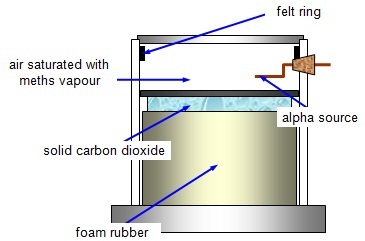- The bottom of the chamber is cooled by solid carbon (V) oxide to around -80° C and the alcohol vapour from the felt ring spreads downwards.
- It is cooled below its normal condensing temperature. As a particle enters the chamber it ionizes the air in its path and alcohol condenses around the path to form millions of tiny alcohol droplets leaving a trail visible because it reflects light from the source.
- Alpha particles leave a thick, short straight tracks. Beta particles leave thin irregular tracks.
- Gamma particles do not produce tracks and since they eject electrons from atoms the tracks are similar to those of beta particles.

### Activity and Half-Life of Elements

The activity of a sample of radioactive element is the rate at which its constituent nuclei decay or disintegrate..
- It is measured in disintegrations per second or Curie (Ci) units, where 1 Ci = 3.7 × 1010 disintegrations per second. 1 micro Curie (u C) = 3.7 × 104 disintegrations per second.
- The law of radioactive decay states that "the activity of a sample is proportional to the number of undecayed nuclei present in the sample".
- The half-life of a radioactive element is the time required for its one-half of the sample to decay.
- It is important to note that although the activity approaches zero, it never goes to zero.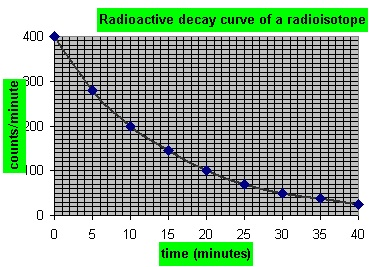#### Examples

1. The half-life of a sample of a radioactive substance is 98 minutes. How long does it take for the activity of the sample to reduce to 1/16th of the original value?2. An isotope has a half-life of 576 hours. Complete the following table and show how mass varies with time from an initial mass of 1280 g?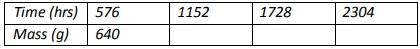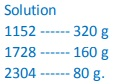3. The initial number of atoms in a sample is 5.12 × 1020. If the half-life of the sample is 3.0 seconds, determine the number of atoms that will have decayed after six seconds.
Solution
After the first half-life, then ½(5.12 × 1020) = 2.56 × 1020 will have decayed.
The second half-life, then ½ (2.56 × 1020) = 1.28 × 1020 will have decayed.
The total number of decayed atoms = (2.56 + 1.28) × 1020 = 3.84 × 1020 atoms.

4. A radioactive element has an initial count rate of 2,400 counts per minute on a scaler.
The count rate falls to 300 units per minute in 30 hours,
a) Calculate the half-life of the element
b) If the initial number of atoms in another sample of the same element is 6.0 × 1020, how many atoms will have decayed in 50 hours?
Solution
a) 2,400 × ½ × ½ × ½ = 300
Three half-lives have a total of 30 hours, thus half-life = 30 /3 = 10 hours
b) Since half-life = 10 hrs half-lives in 50 hrs = 50/10 = 5 hrs.
So the remaining undecayed atoms are ½ × ½ × ½ × ½ × ½ × 6.0 × 1020
= 0.1875 × 1020, thus
The number of atoms which have decayed = (6.0 – 0.1875) × 1020
= 5.812 × 1020

## Nuclear Equations

- Particles making an atom can be written using upper and lower subscripts where a proton, 'p' with charge +1 and mass 1ᴜ, is written as11p.
- A neutron 'n' with no charge but with mass 1u, is written as 10n, while an electron with a charge of -1 and negligible mass is written as 0-1n.
- It is important to note that the principles of conservation apply in radioactive decay.
- That means that the total number of nucleons (neutrons + protons) must be the same before and after decay.
- The L.H.S of the equation must be equal to the R.H.S for both total mass and charge.

### Effects of Radioactive Decay on the Nucleus

#### Alpha decay

A nucleus emitting an alpha particle reduces its mass by 4 atomic mass units and its proton number by 2. The equation can be written as follows: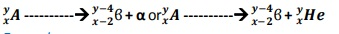Example
Uranium-235 [23592u] changes to Thorium [23190Th] by emitting an alpha particle. Write a nuclear equation to represent the decay.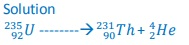The change of an element (nucleus) to another is called transmutation.

#### Beta decay

- The beta particle is an electron.
- Beta particles are produced by changing a neutron to a proton and later to an electron as shown: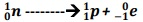- The electron is then ejected from the nucleus and the number of protons increases by 1 while the mass number remains the same (an electron is of negligible mass).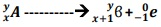Examples
1. Thorium23190Th changes to Proctanium (Po) with the emission of a beta particle. Show the decay using nuclear equation.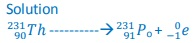2. Write an equation to show how a radioactive isotope of cobalt 6027Co undergoes a beta decay followed by the emission of gamma rays to form a new nuclide X.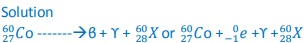3. A radioactive carbon-14 decays to nitrogen by emitting a beta particle as shown.
Determine the values of 'x' and 'y' in the equation below.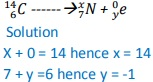#### Other examples

Balance the following equations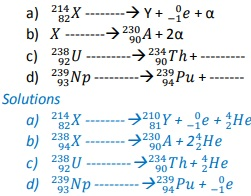### Nuclear Fission

- Nuclear fission is a process in which a nucleus splits into two or more lighter nuclei.
- This process generates large amounts of energy together with neutron emission.
- Nearly 80% of the energy produced appears as kinetic energy of the fission fragments.
- For example Uranium-235 undergoes nuclear fission when bombarded with slow neutrons releasing 2-3 neutrons per Uranium molecule and every neutron released brings about the fission of another Uranium-235 nuclei.
- Another substance which undergoes the same process is Plutonium-239.
- Substances which undergo fission directly with slow neutrons are known as fissile substances or isotopes.

#### Applications of nuclear fission

1. They are used in the manufacture of atomic bombs where tremendous amount of energy is released within a very short time leading to an explosion.
2. When this release of energy is controlled such that it can be released at a steady rate then it is converted into electrical energy hence the principle in nuclear reactors.

### Nuclear Fusion

Nuclear fusion is the thermal combining of light elements to form relatively heavier nuclei.
The process requires very high temperatures for the reacting nuclei to combine upon collision.
These temperatures are provided by ordinary fission bombs. These reactions sometimes known as thermonuclear reactions.
A fusion reaction releases energy at the rate of 3-23 MeV per fusion event i.e. two deuterium (heavy hydrogen) nuclei to form helium.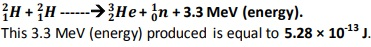#### Application of Nuclear Fusion

1. Used in the production of hydrogen bomb. Possible reactions for an hydrogen bomb include: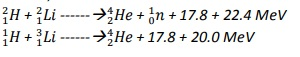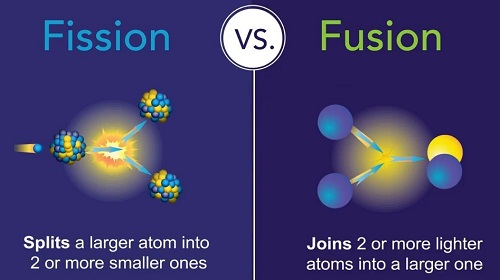#### 1. Carbon dating

- Through the identification of carbon-14 and carbon-12 absorbed by dead plants and animals.
- Scientists can be able to estimate the age of a dead organism.
- Since carbon is a radioactive element with a half-life of 5,600 years archaeologists can be able to estimate the ages of early life through carbon dating.

#### 2. Medicine

- Radiation is used in the treatment of cancer, by using a radioactive cobalt-60 to kill the malignant tissue.
- Radiations are used in taking x-ray photographs using cobalt-60.
- Radiations are used to sterilize surgical instruments in hospitals.
- Radioactive elements can also be used as tracers in medicine where they determine the efficiency of organisms such as kidneys and thyroid glands.

#### 3. Biology and agriculture

- Radioactive sources are used to generate different species of plants with new characteristics that can withstand diseases and drought.
- Insects are sterilized through radiation to prevent the spread of pests and diseases.
- Potatoes exposed to radiation can be stored for a long time without perishing.

#### 4. Industry

- Thickness of metal sheets is measured accurately using radiation from radioactive sources. Recently the manufacture of industrial diamonds is undertaken through transmutation.

#### 5. Energy Source

- In North America, Europe and Russia nuclear reactors are used to generate electricity.
- The amount of fuel used is quite small hence an economical way of generating electricity energy as compared to H.E.P generation.